# Round Price to Nearest Dollar or Cent in Excel & Google Sheets

This tutorial will demonstrate how to round a price to the nearest dollar or cent in Excel and Google Sheets.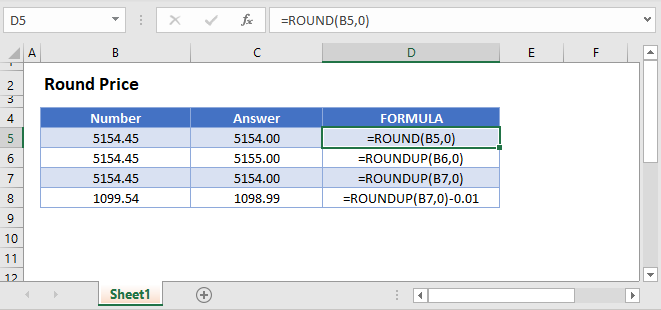## Round Price to Nearest Dollar

There are several rounding functions that you can use to round prices. We will walk through each function below, but they all work the same way; Input num_digits = 0 input to round to the nearest dollar.

### ROUND Function

The ROUND Function can round a currency value to the nearest dollar using standard rounding rules (anything below \$0.50 rounds down, otherwise rounds up).

``=ROUND(B3,0)``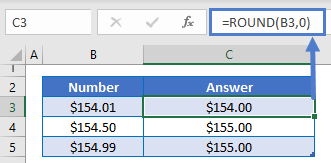### ROUNDUP Function

The ROUNDUP Function will round up to the nearest dollar.

``=ROUNDUP(B3,0)``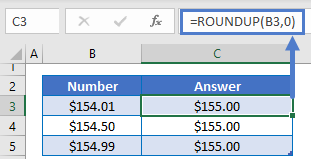### ROUNDDOWN Function

The ROUNDDOWN Function will round down to the nearest dollar.

``=ROUNDDOWN(B3,0)``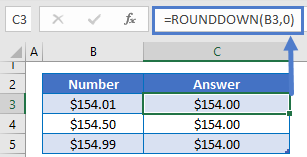## Round Price to Nearest Cent

By setting the num_digits input to 2 we can round to the nearest cent.

### ROUND Function

``=ROUND(B3,2)``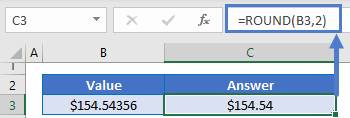### Easy Excel Automation### ROUNDUP Function

``=ROUNDUP(B3,2)``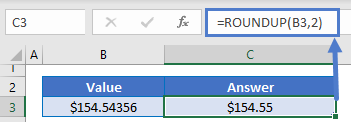### ROUNDDOWN Function

``=ROUNDDOWN(B3,2)``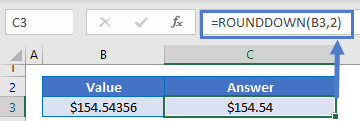## Round Price to end in 99

By subtracting .01 from any of the above formulas, we can round a price to end in 99 cents.

``=ROUND(B3,0)-0.01``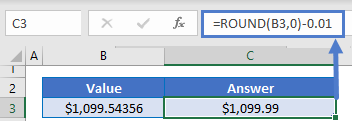## Round Price to Nearest Dollar or Cent in Google Sheets

All of the above examples work exactly the same in Google Sheets as in Excel.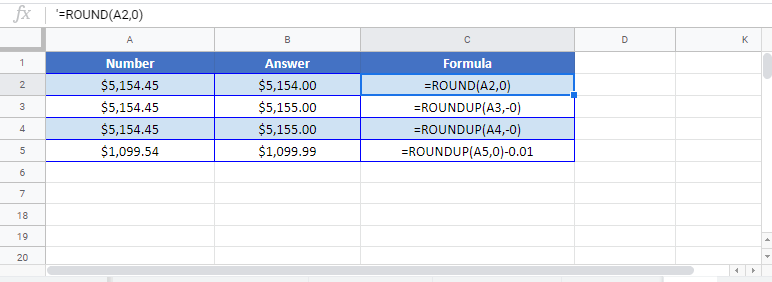### Excel Practice Worksheet

Practice Excel functions and formulas with our 100% free practice worksheets!